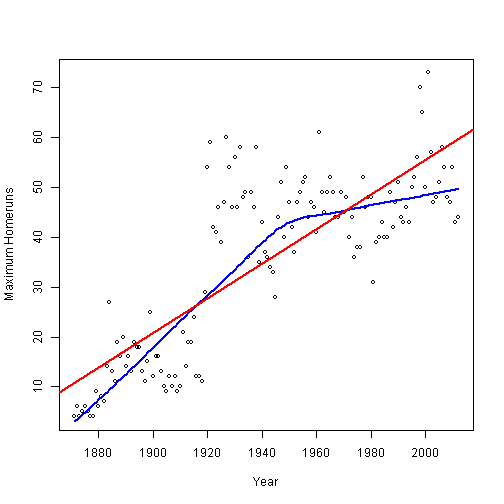Label {Lahman} R Documentation

## Extract the Label for a Variable

### Description

Extracts the label for a variable from one or more of the `*Labels` files.

### Usage

```Label(var, labels = rbind(battingLabels, pitchingLabels, fieldingLabels))
```

### Arguments

 `var` name of a variable `labels` label table(s) to search, a 2-column dataframe containing variable names and labels.

### Value

Returns the variable label, or `var` if no label is found

### Author(s)

Michael Friendly

`battingLabels`, `pitchingLabels`, `fieldingLabels`

### Examples

``````
require(plyr)
# find and plot maximum number of homers per year
batHR <- ddply(subset(Batting, !is.na(HR)), .(yearID),
summarise, max=max(HR))

with(batHR, {
plot(yearID, max,
xlab=Label("yearID"), ylab=paste("Maximum", Label("HR")),
cex=0.8)
lines(lowess(yearID, max), col="blue", lwd=2)
abline(lm(max ~ yearID), col="red", lwd=2)
})
````````````
``````

[Package Lahman version 2.0-1 Index]